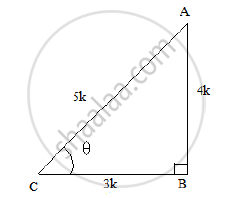# If Tan θ = 4/3, Show that (Sintheta + Cos Theta )=7/5 - Mathematics

If tan θ = 4/3, show that (sintheta + cos theta )=7/5

#### Solution

Let us consider a right ΔABC, right angled at B and ∠𝐶 = 𝜃
Now, we know that tan 𝜃 = (AB)/(BC) = 4/3So, if BC = 3k, then AB = 4k, where k is a positive number.
Using Pythagoras theorem, we have:

AC^2 = AB^2 + BC^2 = (4K)^2 + (3K)^2
⟹ AC^2 = 16K^2 + 9K^2 = 25K^2
⟹ AC = 5k
Finding out the values of sin 𝜃 𝑎𝑛𝑑 cos 𝜃 𝑢𝑠𝑖𝑛𝑔 𝑡ℎ𝑒𝑖𝑟 𝑑𝑒𝑓𝑖𝑛𝑖𝑡𝑖𝑜𝑛𝑠, 𝑤𝑒 ℎ𝑎𝑣𝑒:
Sin 𝜃 = (AB)/(AC) = (4K)/(5K)=4/5

Cos theta= (BC)/(AC) =(3K)/(5K)=3/5

Substituting these values in the given expression, we get:

(sin theta + cos theta )=(4/5 +3/5)=(7/5) = RHS

i.e., LHS = RHS
Hence proved.

Concept: Trigonometric Ratios and Its Reciprocal
Is there an error in this question or solution?

#### APPEARS IN

RS Aggarwal Secondary School Class 10 Maths
Chapter 5 Trigonometric Ratios
Q 18